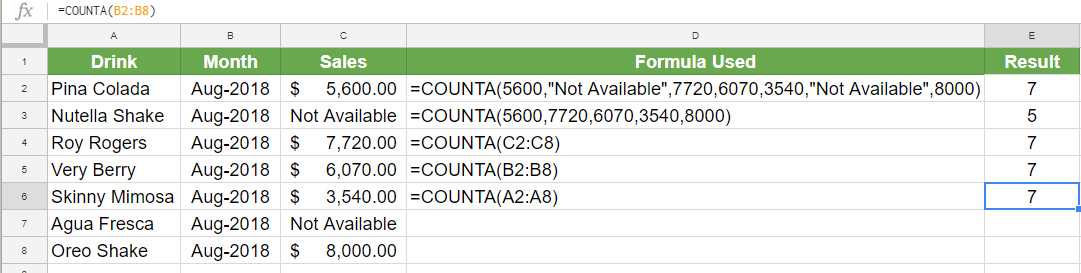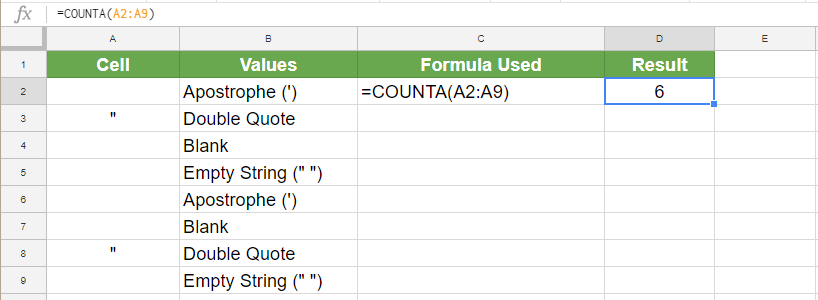Get instant live expert help with Excel or Google Sheets“My Excelchat expert helped me in less than 20 minutes, saving me what would have been 5 hours of work!”

#### Post your problem and you’ll get expert help in seconds.

Your message must be at least 40 characters
Our professional experts are available now. Your privacy is guaranteed.

# How to Use the COUNTA Function in Google Sheets

The COUNTA function in Google sheets is one of the simplest and most used functions. It is very similar to COUNT in syntax and use. The Google Sheets COUNTA function counts the total number of values within a range. In this tutorial, you will learn how to use the COUNTA function in Google Sheets.

## Use the COUNTA function in Google Sheets

### Syntax of COUNTA

COUNTA(value1, [value2, …])

### value 1

This value is required. It is the value or cell reference of the range you want to count.

### [value 2,..]

This value is optional. Additional values or range references to count. Although COUNTA can take a maximum of 30 arguments, Google Sheets allows an arbitrary number of arguments for COUNTA.

### Notes

• COUNTA Google Sheets function can be used to count numbers, dates, formulas, logical expressions, text, errors (e.g., #NUM), etc.
• Google Sheets COUNTA function can count cells containing a leading apostrophe (‘) without any other data in them. Google considers text following the apostrophe (‘) as texts.
• Google Spreadsheets COUNTA can also count cells containing empty strings (“ “) which look empty.
• COUNT and COUNTA are the same functions with one major difference: the ability of COUNTA to count text values in spreadsheets which COUNT cannot do. However, none of them can count blank cells.
• When you modify the content in the range used as the arguments of a COUNTA function, Google Sheets recalculates the result.
• If a value in a range used as an argument for the Google Sheets COUNTA function, the repeated value will be calculated each time despite being repeated.

### Applying COUNTA function in Google Sheets examples

The following examples contain sales data for different drinks for the month of August. It includes the name of the drinks, month and sales.Column D contains the formulas used, and Column E has the results for the corresponding formulas. The formulas in use here are:

`=COUNTA(5600,"Not Available",7720,6070,3540,"Not Available",8000)`

The formula above explicitly counts the values 5600, 7720, 6070,3540, 8000 and “Not Available”. It counts the value “Not Available” twice though it is a repeated value. The main difference between COUNT and COUNTA can be seen from this example. The same arguments provided to the COUNT function would return the value 5, whereas COUNTA counts the text values as well and returns 7.

`=COUNTA(5600,7720,6070,3540,8000)`

Here COUNTA counts the numbers 5600, 7720, 6070,3540, 8000 and returns the number 7.

`=COUNTA(C2:C8)`

Counts both the numeric and text values between C2 to C8. Returns the number 7 which is the count between the values in cell C2 to C8.

`=COUNTA(B2:B8)`

Cell E5 counts the date values in the range B2 to B8. The B column is formatted as dates and COUNTA calculates and returns the total which is 7.

`=COUNTA(A2:A8)`

This formula counts the values from cells A2 to A8. Cells A2 to A8 have text values which can only be counted using the COUNTA Google Sheets function. This formula returns the number 7.

### COUNTA Function with Blanks

COUNTA Google Sheets recognizes text, number, date, and other values but fails to calculate blank cells. In the following example, you will see some cases of how Google Sheets COUNTA function deals with cells containing empty or blank cells.The following example has some empty strings (“ “), double quote (“), apostrophe (‘) and blank values in cells A2 to A9. Column B has the corresponding values in column A described in words. Column C contains the formulas used, and column D has the results.

`=COUNTA(A2:A9)`

This formula counts the empty strings (“ “), double quote (“ “) and apostrophe (‘) values between cells A2 to A9. However, it ignores the blank cells A4 and A7. It returns the result 6 in cell D2.

COUNTA Google Sheets function is one of the most popular functions to count values. Though COUNTA is almost identical to COUNT, the ability to calculate text values has made it more popular than its counterpart. It still has some limitations, like the inability to count blank cells. Apart from that, Google Sheets COUNTA function is an excellent choice for counting values in a range.

Still need some help with Excel formatting or have other questions about Excel? Connect with a live Excel expert here for some 1 on 1 help. Your first session is always free.

### Did this post not answer your question? Get a solution from connecting with the expert.Another blog reader asked this question today on Excelchat:
Related blogs
Solution examplesI want to create a double bar graph from an existing pivot table, but excel won't let me simply add a new field for the column labels and manually put in numbers. What can I do?
Solved by C. F. in 18 minsI have many cells where value is seperated by commas e.g- 1,5,7,8,9 3,0,4,7,8,9,52, Just want to count total value, in the above case it should be 12
Solved by M. J. in 20 minsI have three spreadsheets with names of registered people for an event. I need to combine them and then filter so that I know if any of the people in sheet 1 are also listed on sheet 2 and sheet 3
Solved by C. A. in 40 minsI have a column of percentages (11 in a column). I want to have a percentage at the bottom of the column that tells me how many of the entries are = to 100%.
Solved by X. E. in 20 minsHi, Hopefully someone will be able to help me. I'm wanting to calculate the average sum contained within a range of cells discarding blanks. The problem that I'm encountering is that there is an additional feature where I only want to take into consideration columns that contain specific text. =SUMIF(G79:HG79,"Sep-18",(G6:HG6/COUNTA(G6:HG6))) G79:HG79 will contain the specific text with G:HG containing the digits. Cheers all in advance
Solved by M. J. in 20 mins## Subscribe to Excelchat.coAnother blog reader asked this question today on Excelchat: